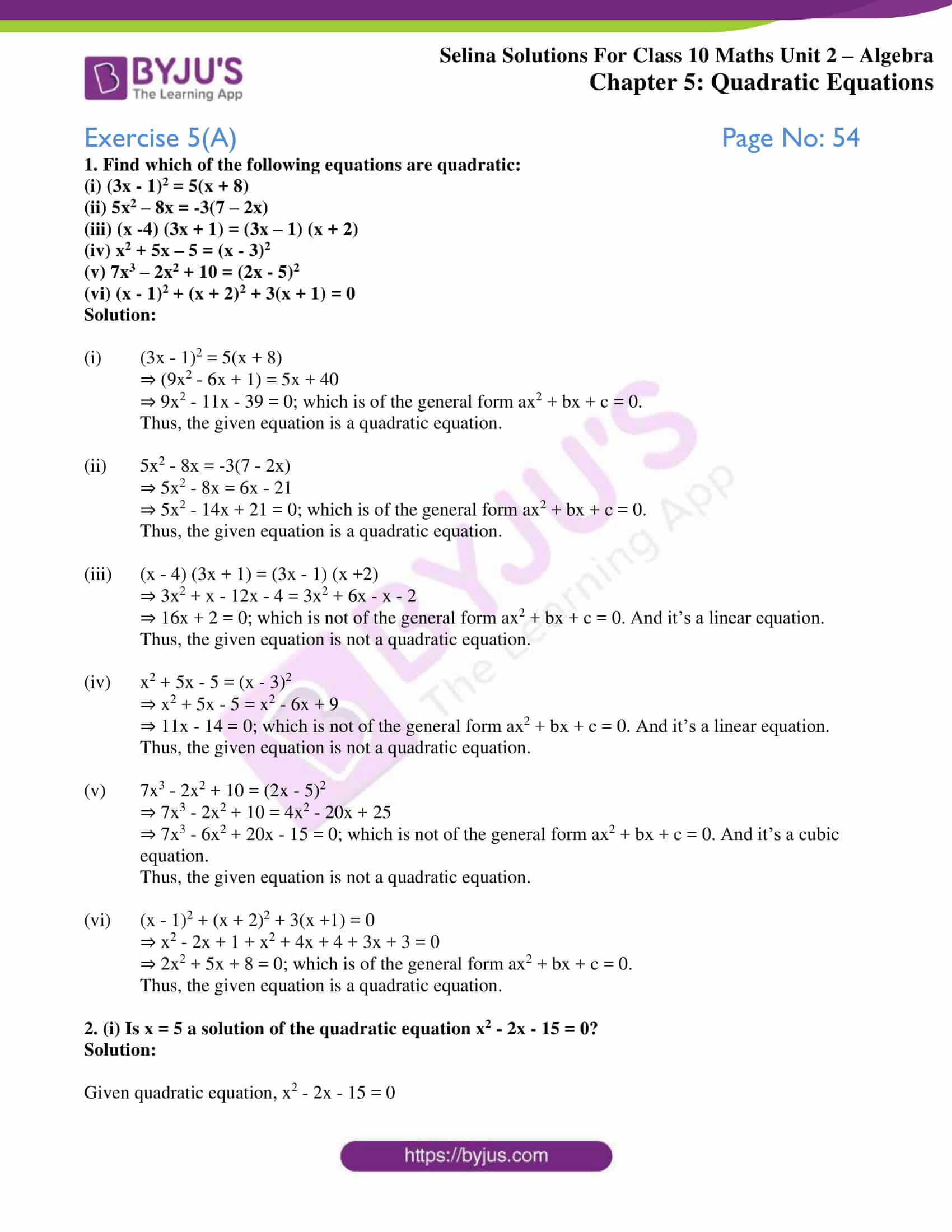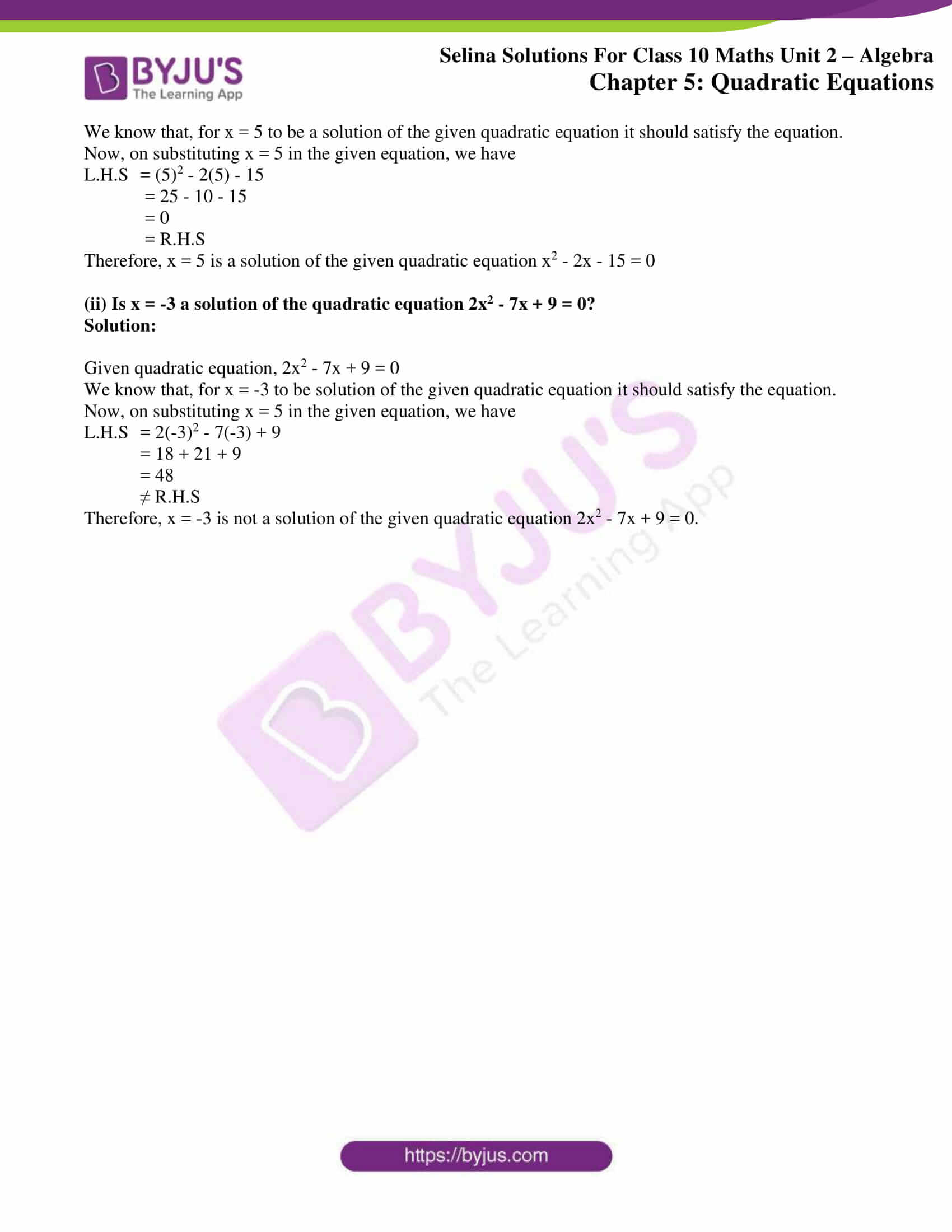# Selina Solutions Concise Maths Class 10 Chapter 5 Quadratic Equations Exercise 5(A)

Quadratic equations are widely used in Mathematics. In this exercise, the students will be able to identify quadratic equations and also verify the solution of a quadratic equation. The Selina Solutions for Class 10 Maths is the best resource students can rely on for learning the correct methods to solve these problems. The solutions of this exercise are available in PDF format in the Concise Selina Solutions for Class 10 Maths Chapter 5 Quadratic Equations Exercise 5(A) PDF in the links below.### Access Selina Solutions Concise Maths Class 10 Chapter 5 Quadratic Equations Exercise 5(A)

1. Find which of the following equations are quadratic:

(i) (3x – 1)2 = 5(x + 8)

(ii) 5x2 – 8x = -3(7 – 2x)

(iii) (x -4) (3x + 1) = (3x – 1) (x + 2)

(iv) x2 + 5x – 5 = (x – 3)2

(v) 7x3 – 2x2 + 10 = (2x – 5)2

(vi) (x – 1)2 + (x + 2)2 + 3(x + 1) = 0

Solution:

(i) (3x – 1)2 = 5(x + 8)

⇒ (9x2 – 6x + 1) = 5x + 40

⇒ 9x2 – 11x – 39 = 0; which is of the general form ax2 + bx + c = 0.

Thus, the given equation is a quadratic equation.

(ii) 5x2 – 8x = -3(7 – 2x)

⇒ 5x2 – 8x = 6x – 21

⇒ 5x2 – 14x + 21 = 0; which is of the general form ax2 + bx + c = 0.

Thus, the given equation is a quadratic equation.

(iii) (x – 4) (3x + 1) = (3x – 1) (x +2)

⇒ 3x2 + x – 12x – 4 = 3x2 + 6x – x – 2

⇒ 16x + 2 = 0; which is not of the general form ax2 + bx + c = 0. And it’s a linear equation.

Thus, the given equation is not a quadratic equation.

(iv) x2 + 5x – 5 = (x – 3)2

⇒ x2 + 5x – 5 = x2 – 6x + 9

⇒ 11x – 14 = 0; which is not of the general form ax2 + bx + c = 0. And it’s a linear equation.

Thus, the given equation is not a quadratic equation.

(v) 7x3 – 2x2 + 10 = (2x – 5)2

⇒ 7x3 – 2x2 + 10 = 4x2 – 20x + 25

⇒ 7x3 – 6x2 + 20x – 15 = 0; which is not of the general form ax2 + bx + c = 0. And it’s a cubic equation.

Thus, the given equation is not a quadratic equation.

(vi) (x – 1)2 + (x + 2)2 + 3(x +1) = 0

⇒ x2 – 2x + 1 + x2 + 4x + 4 + 3x + 3 = 0

⇒ 2x2 + 5x + 8 = 0; which is of the general form ax2 + bx + c = 0.

Thus, the given equation is a quadratic equation.

2. (i) Is x = 5 a solution of the quadratic equation x2 – 2x – 15 = 0?

Solution:

Given quadratic equation, x2 – 2x – 15 = 0

We know that, for x = 5 to be a solution of the given quadratic equation it should satisfy the equation.

Now, on substituting x = 5 in the given equation, we have

L.H.S = (5)2 – 2(5) – 15

= 25 – 10 – 15

= 0

= R.H.S

Therefore, x = 5 is a solution of the given quadratic equation x2 – 2x – 15 = 0

(ii) Is x = -3 a solution of the quadratic equation 2x2 – 7x + 9 = 0?

Solution:

Given quadratic equation, 2x2 – 7x + 9 = 0

We know that, for x = -3 to be solution of the given quadratic equation it should satisfy the equation.

Now, on substituting x = 5 in the given equation, we have

L.H.S = 2(-3)2 – 7(-3) + 9

= 18 + 21 + 9

= 48

≠ R.H.S

Therefore, x = -3 is not a solution of the given quadratic equation 2x2 – 7x + 9 = 0.